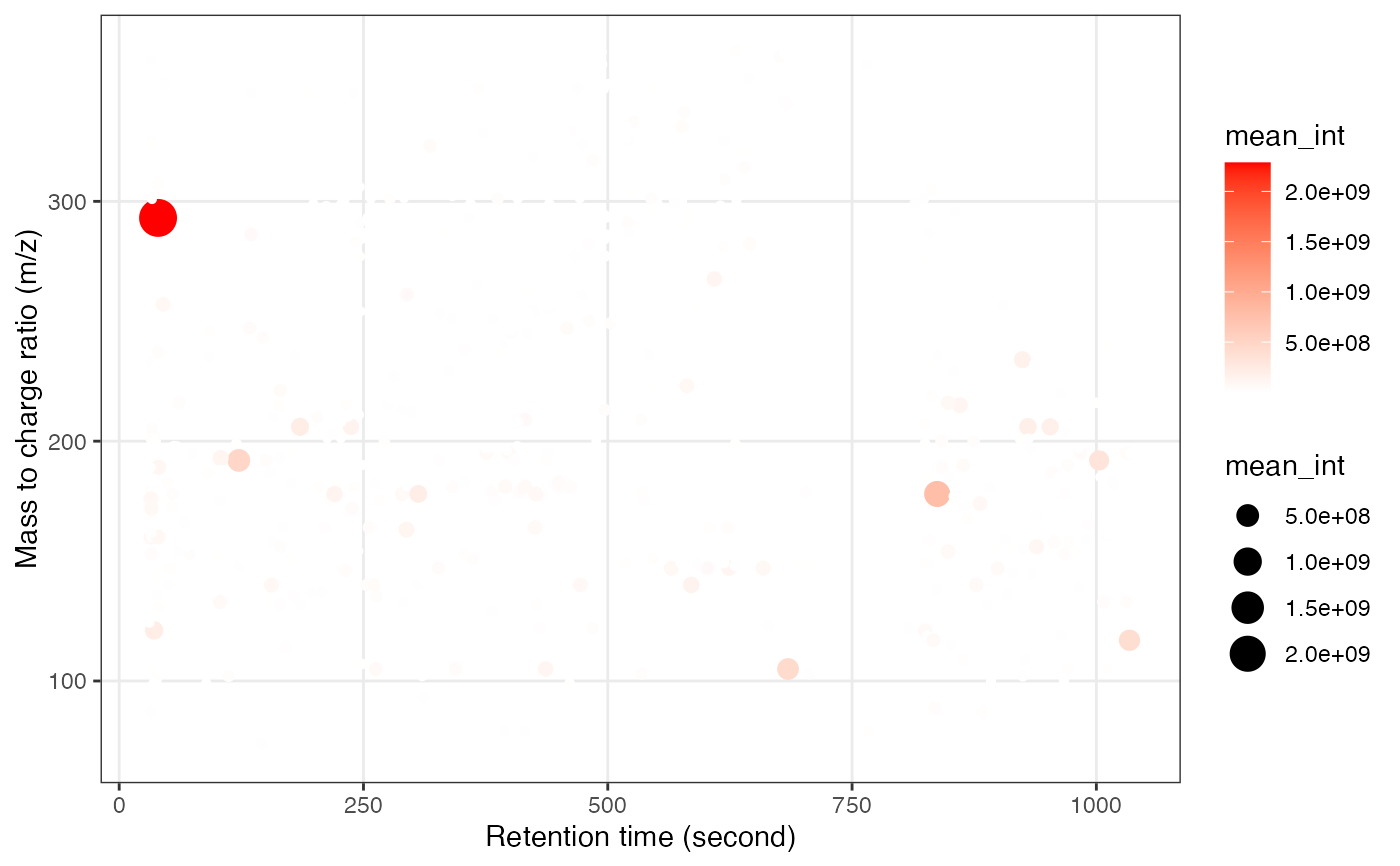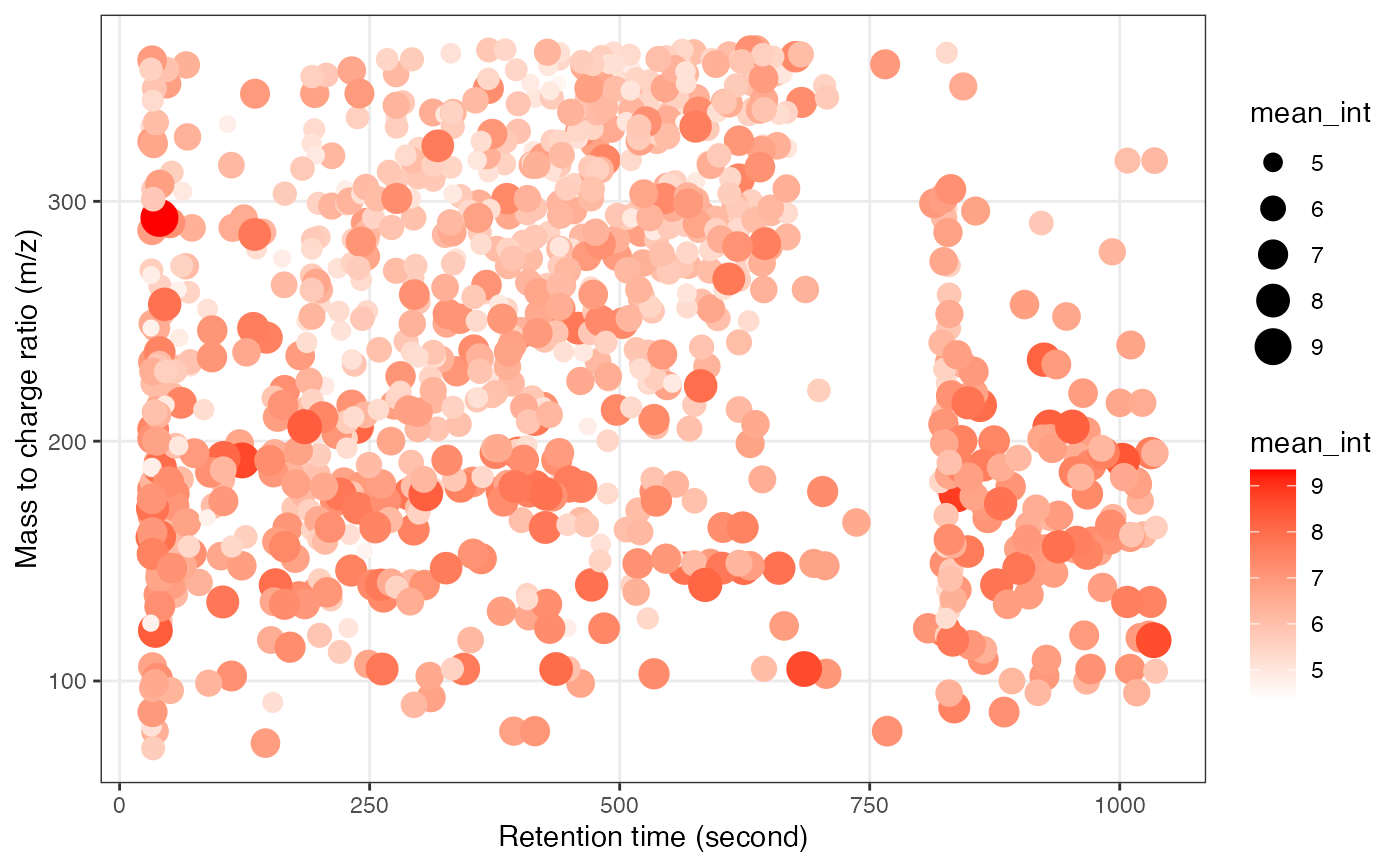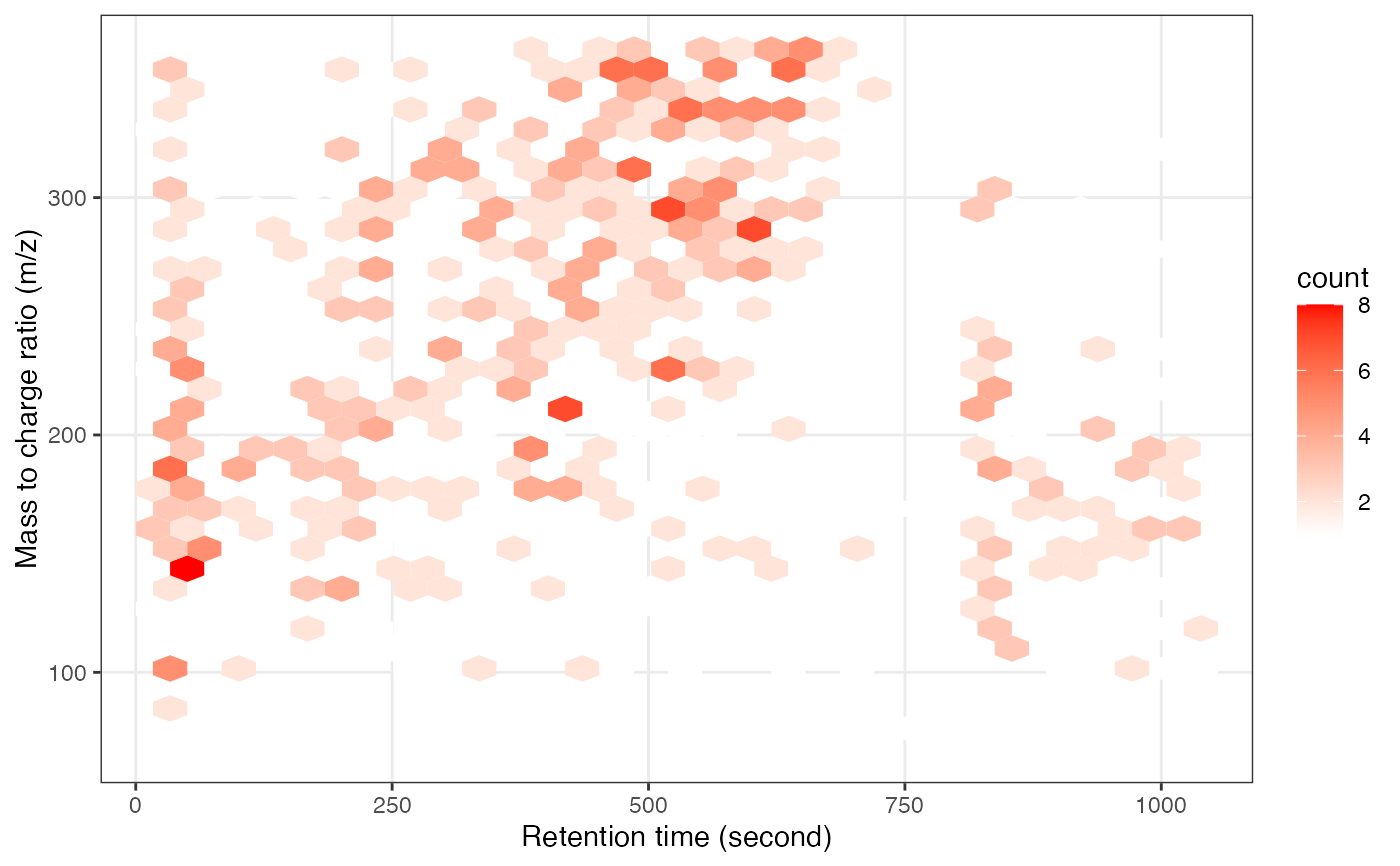mz and rt plot of one mass_dataset class object.

show_mz_rt_plot(object, hex = FALSE)

## Arguments

object

(required) mass_dataset class object.

hex

hex or not.

A ggplot2 object

## Author

Xiaotao Shen shenxt1990@outlook.com

## Examples

data("expression_data")
data("sample_info")
data("variable_info")

object =
create_mass_dataset(
expression_data = expression_data,
sample_info = sample_info,
variable_info = variable_info,
)

show_mz_rt_plot(object)show_mz_rt_plot(log(object, 10))show_mz_rt_plot(object, hex = TRUE)